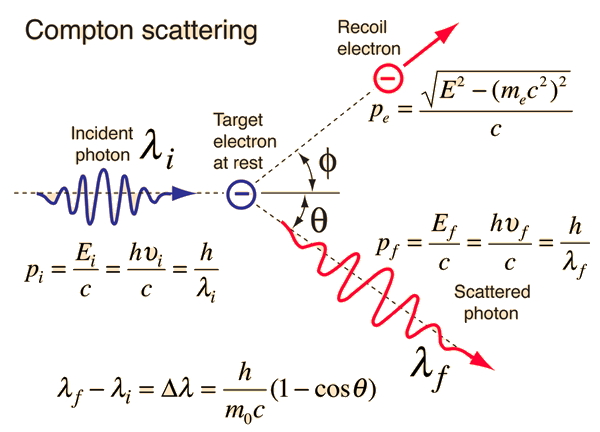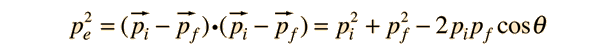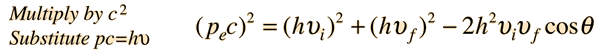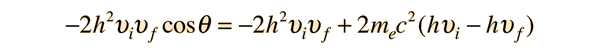# Compton Scattering EquationIn his explanation of the Compton scattering experiment, Arthur Compton treated the x-ray photons as particles and applied conservation of energy and conservation of momentum to the collision of a photon with a stationary electron. Using the Planck relationship and the relativistic energy expression, conservation of energy takes the formConservation of momentum requireswhere p=E/c is used for the photon momentum. Squaring this equation using the scalar product givesAgain using the Planck relationship and the relativistic energy expression:The energy conservation expression above can be squared to giveThese two forms can be equated to givewhich can be rearranged toand finally to the standard Compton formula:Calculation Compton scattering experiment
Index

References:
Compton

Blatt
Sec 4.6

 HyperPhysics***** Quantum Physics R Nave
Go Back